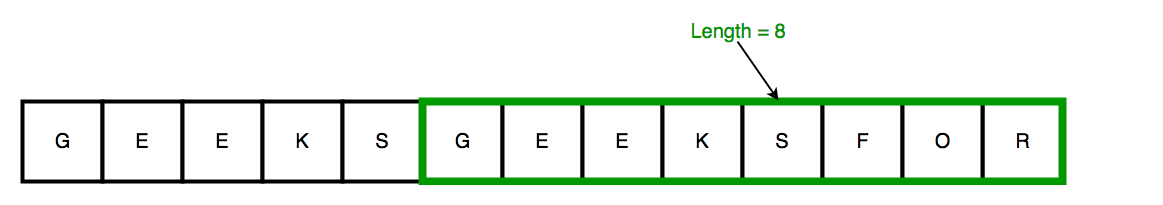# Length of the smallest sub-string consisting of maximum distinct characters

Given a string of length N, find the length of the smallest sub-string consisting of maximum distinct characters. Note : Our output can have same character.Examples:

```Input : "AABBBCBB"
Output : 5

Input : "AABBBCBBAC"
Output : 3
Explanation : Sub-string -> "BAC"

Input : "GEEKSGEEKSFOR"
Output : 8
Explanation : Sub-string -> "GEEKSFOR"```

Method 1 (Brute Force)

We can consider all sub-strings one by one and check for each sub-string both conditions together
1. sub-string%u2019s distinct characters is equal to maximum distinct characters
2. sub-sting%u2019s length should be minimum .
Time Complexity : O(n^3)

Implementation:
/* C++ program to find the length of the smallest
substring consisting of maximum distinct characters */
#include <bits/stdc++.h>
using namespace std;
#define NO_OF_CHARS 256
// Find maximum distinct characters in any string
int max_distinct_char(string str, int n){
// Initialize all character's count with 0
int count[NO_OF_CHARS] = {0};
// Increase the count in array if a character
// is found
for (int i = 0; i < n; i++)
count[str[i]]++;
int max_distinct = 0;
for (int i = 0; i < NO_OF_CHARS; i++)
if (count[i] != 0)
max_distinct++;
return max_distinct;
}
int smallesteSubstr_maxDistictChar(string str){
int n = str.size(); // size of given string
// Find maximum distinct characters in any string
int max_distinct = max_distinct_char(str, n);
int minl = n; // result
// Brute force approach to find all substrings
for (int i=0 ;i<n ;i++){
for (int j=0; j<n; j++){
string subs = str.substr(i,j);
int subs_lenght = subs.size();
int sub_distinct_char = max_distinct_char(subs, subs_lenght);
// We have to check here both conditions together
// 1. substring's distinct characters is equal
// to maximum distinct characters
// 2. substing's length should be minimum
if (subs_lenght < minl && max_distinct == sub_distinct_char){
minl = subs_lenght;
}
}
}
return minl;
}
/* Driver program to test above function */
int main()
{
// Input String
string str = "AABBBCBB";
int len = smallesteSubstr_maxDistictChar(str);
cout << " The length of the smallest substring"
" consisting of maximum distinct "
"characters : " << len;
return 0;
}
Output:
``` The length of the smallest substring consisting
of maximum distinct characters : 5
```#### More Articles of M Mounika:

Name Views Likes
C++ Segmented Sieve (Print Primes In a Range) 162 0
C++ Sieve Of Erastosthenes 135 0
C++ Gold Mine Problem 295 0
C++ Merge K Sorted Arrays 117 0
C++ K Centers Problem 240 0
C++ Find Nth Catalan Number 311 0
C++ Inplace Rotate square matrix by 90 degrees 285 0
C++ Find Non Repeating Elements in Array 87 0
C++ Merge Two Binary Trees 121 0
C++ Sum of Numbers From Root To Leaf Paths 89 0
C++ Meta Strings 91 0
C++ Flood Fill Algorithm 402 0
C++ smallest substring with maximum distinct characters 200 0
C++ Smallest window with all characters in string 93 0
C++ Minimum Removal of Characters from string to make its permutation as palindrome 87 0
C++ Minimum characters added at front of string in palindrome conversion 69 0
C++ Number of Bracket Reversals needed to make expression Balanced 72 0
C++ String to Palindrome with Append Function 83 0
C++ WildCard pattern matching 76 0
C++ Anagram substring Search 72 0
C++ Manachars Algorithm 74 0
C++ Search String in Grid 83 0
C++ String Matching(Z Algorithm) 67 0
C++ String Matching(Naive Algorithm) 113 0
C++ String Matching(KMP Algorithm) 140 0
C++ Remove Duplicates From String 110 0
C++ Basics of String Manipulation 85 1
C++ Disjoint Data Structure Cycle Detection 87 0
C++ Problem On Disjoint Data Structures 95 0
C++ Disjoint Data Structures Part3 79 0
Disjoint Data Structures Part2 90 0
Disjoint Data Structures 93 1
C++ Segment Trees 321 2
C++ Trie Cost of Data 290 1
C++ Trie Datastructure 279 1
C++ Greedy Approach Minimum number of coins 526 0
C++ Greedy Approach Maximum height Pyramid 328 1
C++ Greedy Approach String lexicographically largest subsequence 247 0
C++ Greedy Approach Lexicographically largest subsequence 364 0
C++ Greedy Approach Prims MST 398 1
C++ Greedy Approach Krushkals MST 458 1
C++ Greedy Approach N-array maximum sum 333 1
C++ Greedy Approach Policemen Catch Thieves 563 1
C++ Greedy Approach Maximum product Subset 546 1
C++ Greedy Approach Minimum Product Subset 349 1
C++ Greedy Approach Fractional Knapsack 737 1
C++ Greedy Approach-Activity Selection Problem 745 1
C++ Greedy Approach-Egyptian Fractions 640 0
C++ Greedy Approach-Huffman Codes 1031 1
C++ Introduction to Greedy Approach 955 2